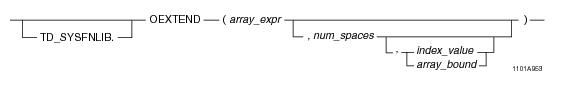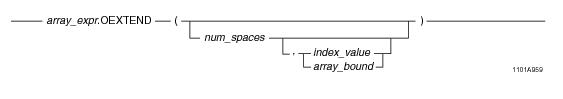# 15.00 - OEXTEND - Teradata Database

## Teradata Database SQL Functions, Operators, Expressions, and Predicates

Product
Release Number
15.00
Content Type
Programming Reference
Publication ID
B035-1145-015K
Language
English (United States)

## OEXTEND

### Purpose

Allocates space for one or more new elements in array_expr.

### Syntax

System function syntax:Method-style syntax:where:

 Syntax element… Specifies… TD_SYSFNLIB the name of the database where the method is located. array_expr one of the following: A reference to an ARRAY column An ARRAY constructor expression. See “ARRAY Constructor Expression” on page 195. A UDF expression A UDM expression num_spaces an optional unsigned integer value representing the number of NULL elements to append to array_expr. index_value an optional unsigned integer value used as an index to an element in array_expr. array_bound an optional array instance of the predefined array type ArrayVec with a comma-separated list of integer values to define the bounds. For details, see “ARRAY Scope Reference” on page 198.

### ANSI Compliance

This is a Teradata extension to the ANSI SQL:2011 standard.

### Usage Notes

OEXTEND takes an array expression as an argument and allocates space for one or more new elements in the array.

 IF you specify... THEN the method... OEXTEND() appends a single NULL element to the ARRAY value. For a multidimensional (n-D) ARRAY, this is done in row-major order. OEXTEND(num_spaces) appends n NULL elements to the ARRAY value, where n = num_spaces. For an n-D ARRAY, this is done in row-major order. OEXTEND(num_spaces, index_value) or OEXTEND(num_spaces, array_bound) appends n copies of the element indexed by either index_value or array_bound to the ARRAY value, where n = num_spaces. For an n-D ARRAY, this is done in row-major order.

The OEXTEND method with zero, one, or two arguments of INTEGER type is compatible with the Oracle EXTEND method, for one-dimensional ARRAY types. However, the empty set of parentheses required when OEXTEND is called with no arguments is a deviation from Oracle syntax.

OEXTEND returns an error in the following cases:

• If array_expr is NULL.
• If either index _value or array_bound is NULL.
• If the number of elements appended by OEXTEND overflows the maximum size of the array.
• If num_spaces is NULL, no error occurs, but no action is taken by OEXTEND.

### Result Type

OEXTEND returns a new modified copy of the array argument.

### Example

Consider the following one-dimensional ARRAY data type and table.

`CREATE TYPE phonenumbers AS VARRAY(20) OF CHAR(10);`
` `
`CREATE TABLE employee_info (eno INTEGER, phonelist phonenumbers);`

The table is populated with the following values:

`/* The first 2 elements are populated; the rest are uninitialized. */`
`INSERT INTO employee_info VALUES (1, `
`   phonenumbers('1112223333', '6195551234'));`
` `
`/* Empty ARRAY instance */`
`INSERT INTO employee_info VALUES (2, `
`   phonenumbers());`
` `
`/* Update empty ARRAY instance such that element 3 is set to a value;`
`   Then elements 1 and 2 are set to NULL, the rest are uninitialized */`
` `
`UPDATE employee_info`
`SET phonelist = '8584850000'`
`WHERE id = 2;`

The following query extends the phonelist array with a single NULL element.

`SELECT eno, phonelist.OEXTEND()`
`FROM employee_info;`

The following is the result of the query.

`ENO         phonelist.OEXTEND()`
`---         -------------------`
` 1           ('1112223333', '6195551234',NULL)`
` 2           (NULL,NULL,'8584850000',NULL)`

The following query extends the phonelist array with three NULL elements.

`SELECT eno, phonelist.OEXTEND(3) `
`FROM employee_info;`

The following is the result of the query.

`ENO         phonelist.OEXTEND(3)`
`---         --------------------`
` 1           ('1112223333', '6195551234',NULL,NULL,NULL)`
` 2           (NULL,NULL,'8584850000',NULL,NULL,NULL)`

The following query extends the phonelist array with two copies of element 1.

`SELECT eno, phonelist.OEXTEND(2,1) `
`FROM employee_info`
`WHERE eno = 1;`

The following is the result of the query.

`ENO         phonelist.OEXTEND(2,1)`
`---         ----------------------`
` 1           ('1112223333', '6195551234', '1112223333', '1112223333')`

The following is the same query using function-style syntax.

`SELECT eno, OEXTEND(phonelist,2,1) `
`FROM employee_info`
`WHERE eno = 1;`

### Example

The following example shows how you can use the OEXTEND method to fill the end of a constructed ARRAY with NULL elements so that these elements are no longer in an uninitialized state. This can be helpful when the ARRAY may be used as an argument to another system function or operator.

`CREATE TYPE myarray AS VARRAY(5) OF INTEGER;`
` `
`CREATE TABLE mytab (id INTEGER, ary myarray);`
` `
`/* Populate the first 3 elements. The last 2 elements are uninitialized. */`
`INSERT INTO mytab VALUES (1, NEW myarray(1,2,3));`
` `
`/* Fill the last 2 elements with NULLs. */`
`UPDATE mytab`
`SET ary = ary.OEXTEND(2)`
`WHERE id = 1;`
` `
`SELECT ary FROM mytab;`

The following is the result of the query.

`(1,2,3,NULL,NULL)`

### Example

Consider the following 2-D ARRAY data type and table.

`CREATE TYPE shot_ary AS VARRAY(1:50)(1:50) OF INTEGER;`
` `
`CREATE TABLE seismic_table (`
`   id INTEGER,`
`   shots shot_ary);`

The table is populated with the following values:

`/* The first 2 elements are populated; the rest are uninitialized. */`
`INSERT INTO seismic_table VALUES (1, shot_ary(11, 12));`
` `
`/* Empty ARRAY instance */`
`INSERT INTO seismic_table VALUES (2, shot_ary());`
` `
`/* Update empty ARRAY instance such that element  is set to a value; Then elements  and  are set to NULL, the rest are uninitialized */`
` `
`UPDATE seismic_table`
`SET shots = 1133`
`WHERE id = 2;`

The following query extends the shots array with a single NULL element.

`SELECT id, shots.OEXTEND() `
`FROM seismic_table;`

The following is the result of the query.

`ID          shots.OEXTEND()`
`--          ---------------`
` 1           (11,12,NULL) `
` 2           (NULL,NULL,1133,NULL)`

The following query extends the shots array with three NULL elements.

`SELECT id, shots.OEXTEND(3) `
`FROM seismic_table;`

The following is the result of the query.

`ID          shots.OEXTEND(3)`
`--          ----------------`
` 1           (11,12,NULL,NULL,NULL) `
` 2           (NULL,NULL,1133,NULL,NULL,NULL) `

The following query extends the shots array with two copies of element .

`SELECT id, shots.OEXTEND(2, NEW arrayVec(1,1)) `
`FROM seismic_table`
`WHERE id = 1;`

The following is the result of the query.

`ID          shots.OEXTEND(2, NEW arrayVec(1,1))`
`--          -----------------------------------`
` 1           (11,12,11,11) `